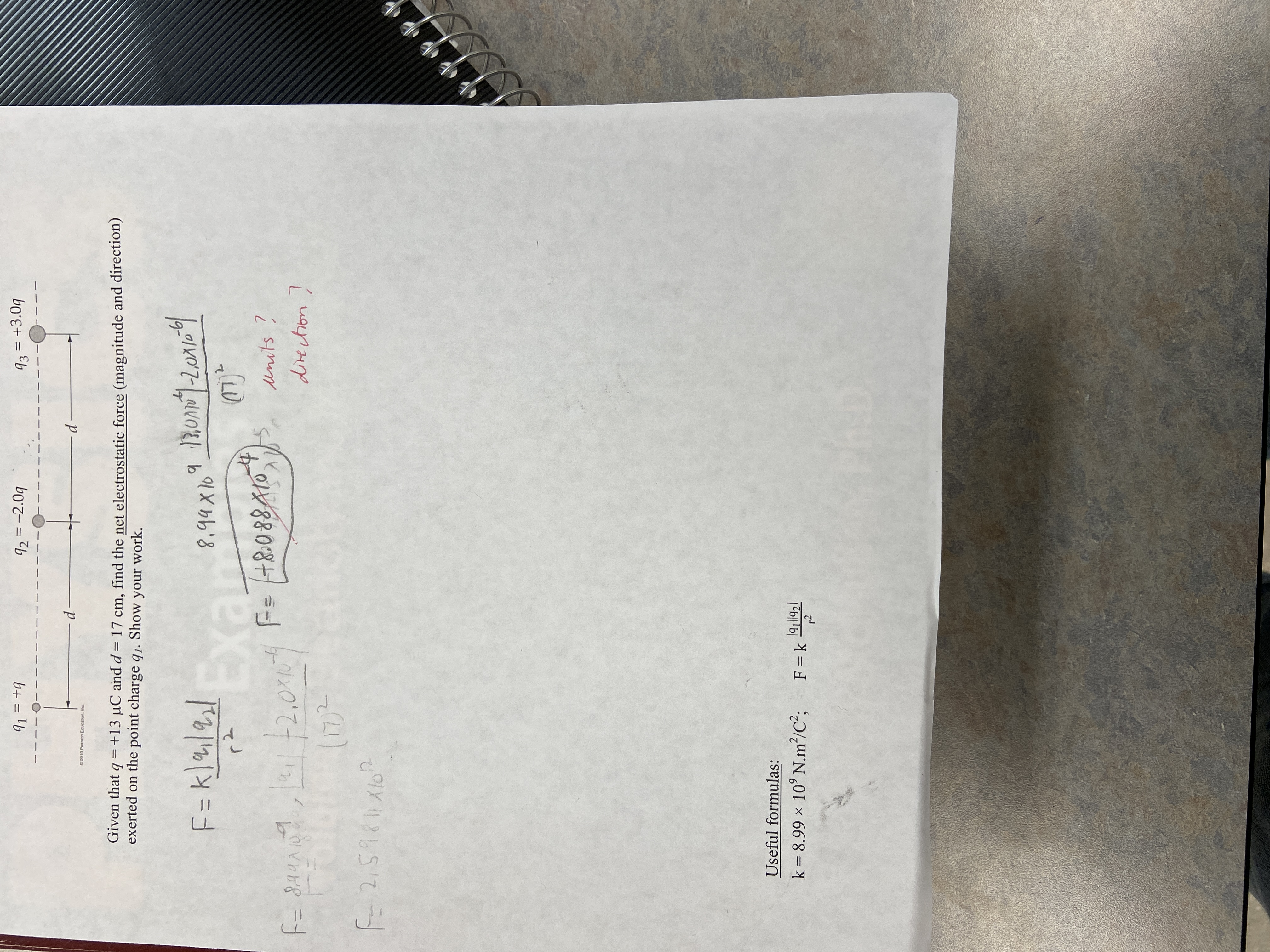# 91 = +992 = -2.0993 = +3.0qO2010 Pearson Education, Inc.Given that q =+13 µC and d= 17 cm, find the net electrostatic force (magnitude and direction)exerted on the point charge q1. Show your work.F=k]/2/ExanF=+8:088A108.99X10r2(17)-tounits ?21/-2,0XF= 8,44Adirection)F-2,59811 XloUseful formulas:k = 8.99 × 10° N.m²/C²;F = kr2

Question
38 views

I got some wrong question how to solve it to find units or directionhelp_outlineImage Transcriptionclose91 = +9 92 = -2.09 93 = +3.0q O2010 Pearson Education, Inc. Given that q =+13 µC and d= 17 cm, find the net electrostatic force (magnitude and direction) exerted on the point charge q1. Show your work. F=k]/2/ Exan F=+8:088A10 8.99X10 r2 (17)- to units ? 21/-2,0X F= 8,44A direction) F-2,59811 Xlo Useful formulas: k = 8.99 × 10° N.m²/C²; F = k r2 fullscreen
check_circle

Step 1

Given:

Charge on q1 = 13×10-6 C

Charge on q2 = -2×13×10-6 C = 26×10-6 C

Charge on q3 = +3×13×10-6 C = 39×10-6 C

Distance between charges (d) = 17 cm = 17×10-2 m.

Step 2
Step 3

### Want to see the full answer?

See Solution

#### Want to see this answer and more?

Solutions are written by subject experts who are available 24/7. Questions are typically answered within 1 hour.*

See Solution
*Response times may vary by subject and question.
Tagged in
SciencePhysics

### Electric Charges and Fields